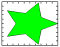# fill

Filled 2-D polygons## Syntax

```fill(X,Y,C) fill(X,Y,ColorSpec) fill(X1,Y1,C1,X2,Y2,C2,...) fill(...,'PropertyName',PropertyValue) fill(ax,...) h = fill(...) ```

## Description

The `fill` function creates colored polygons.

`fill(X,Y,C)` creates filled polygons from the data in `X` and `Y` with vertex color specified by `C`. `C` is a vector or matrix used as an index into the colormap. If `C` is a row vector, `length(C)` must equal `size(X,2)` and `size(Y,2)`; if `C` is a column vector, `length(C)` must equal `size(X,1)` and `size(Y,1)`. If necessary, `fill` closes the polygon by connecting the last vertex to the first. The values in `X` and `Y` can be numeric, datetime, duration, or categorical values.

`fill(X,Y,ColorSpec)` fills the polygons with the color specified by `ColorSpec`, which can be one of the following values:

• A color name or a short name: `'red'` (or `'r'`), `'green'` (or `'g'`), `'blue'` (or `'b'`), `'cyan'` (or `'c'`), `'magenta'` (or `'m'`), `'yellow'` (or `'y'`), `'black'` (or `'k'`), `'white'` (or `'w'`).

• An RGB triplet specified as a 1-by-3 vector whose elements are the intensities of the red, green, and blue components of a color. The intensities must be in the range [0, 1]. For example, `[0.7 0.8 1]` specifies light blue.

`fill(X1,Y1,C1,X2,Y2,C2,...)` specifies multiple two-dimensional filled areas.

`fill(...,'PropertyName',PropertyValue)` allows you to specify property names and values for a patch graphics object.

`fill(ax,...)` creates the polygons in the axes specified by `ax` instead of in the current axes (`gca`). The option `ax` can precede any of the input argument combinations in the previous syntaxes.

`h = fill(...)` returns a vector of patch objects.

## Examples

collapse all

Define the data.

```t = (1/16:1/8:1)'*2*pi; x = cos(t); y = sin(t);```

Create a red octagon using the `fill` function.

```fill(x,y,'r') axis square```## Tips

If `X` or `Y` is a matrix, and the other is a column vector with the same number of elements as rows in the matrix, `fill` replicates the column vector argument to produce a matrix of the required size. `fill` forms a vertex from corresponding elements in `X` and `Y` and creates one polygon from the data in each column.

If `X` or `Y` contains one or more `NaN` values, then `fill` does not fill the polygons.

The type of color shading depends on how you specify color in the argument list. If you specify color using the `ColorSpec` argument, `fill` generates flat-shaded polygons by setting the patch object's `FaceColor` property to the corresponding RGB triplet.

If you specify color using `C`, `fill` scales the elements of `C` by the values specified by the axes property `CLim`. After scaling `C`, `C` indexes the current colormap.

If `C` is a row vector, `fill` generates flat-shaded polygons where each element determines the color of the polygon defined by the respective column of the `X` and `Y` matrices. Each patch object’s `FaceColor` property is set to `'flat'`. Each row element becomes the `CData` property value for the `n`th patch object, where n is the corresponding column in `X` or `Y`.

If `C` is a column vector or a matrix, `fill` uses a linear interpolation of the vertex colors to generate polygons with interpolated colors. It sets the patch graphics object `FaceColor` property to `'interp'` and the elements in one column become the `CData` property value for the respective patch object. If `C` is a column vector, `fill` replicates the column vector to produce the required sized matrix.

## Extended Capabilities

### Topics

Introduced before R2006a